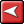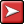## (TD) 6.8 Modeling n-bit Gates## (TD) 6.8 Modeling n-bit GatesNext we will model the DONE signal that we originally drew as an input to the state machine. The DONE signal is generated by performing a bitwise AND of the COUNT signal (we are done whenever all the counter bits are high).

To model the DONE Signal:

1. Double click on the DONE signal name to open the Signal Properties dialog box.

2. Enter the following equation in the Boolean Equation edit box: &COUNT

3. Check the Simulate radio button. The resulting signal should look like the hand drawn signal except that it is a purple simulated signal.

The & operator when used as a unary operator is called a reduction-AND operation. A reduction-AND indicates that all the bits of the input signal should be ANDed together to generate a single bit output. This is equivalent to the following equation: COUNT & COUNT & COUNT & ...

One nice benefit of using a reduction operator instead of the above equation is that it automatically scales the circuit to match the current size of the COUNT signal (it’s also a lot easier to type)!